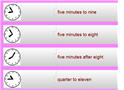# Math Games/Juegos de Matemáticas

• In this fast-paced car racing game, students will practice multiplication facts to 10 times 10.

In this fun basketball game, young students will have fun multiplying one-digit numbers.

In this game students will multiply one-, two-, and three-digit numbers by 5, 6, 7, 8, and 9. Kids can play this game alone or in teams.

In this fast-paced racing game students will use repeated addition to model multiplication problems.

In this fast-paced math game, students will identify and use the commutative, associative, and identity property of multiplication.

In this game students will multiply 2-digit numbers by 1-digit numbers. They can play it alone or in pairs.

In this game students will count various US coins and match the pictures of the coins with the correct amounts.

In this fun soccer game, students will add two-digit numbers to get a chance to kick the ball and score points.

In this interactive soccer game, 2nd grade students will practice adding 2-digit numbers.

In this fun place value game, students must pass the ball to the receiver in order to be given the chance to answer a problem and earn points.Time Matching Game
This game is suitable for 3rd grade students and English language learners of all ages. The object of each problem is to match the analog clocks with the correct phrase.

In this interactive basketball game, 3rd grade students will practice telling time from analog clocks to the nearest minutes.

This is a printable time game that can be used as a classroom activity with elementary students.